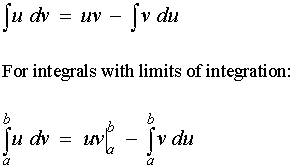# How do you integrate int x^3e^x by integration by parts method?

Feb 2, 2017

Using integration by parts: $= {e}^{x} \left({x}^{3} - 3 {x}^{2} + 6 x - 6\right) + C$

#### Explanation:

The equation for integration by parts is this:I think mathematicians use similar letters on purpose to confuse people. Thats why they chose $u$ and $v$, the worst possible letters.

Anyways, we have to pick our $u$.

let $u = {x}^{3}$ so $\mathrm{du} = 3 {x}^{2} \mathrm{dx}$

That leaves the rest of the integral to be $\mathrm{dv}$

let $\mathrm{dv} = {e}^{x} \mathrm{dx}$ so by taking the integral of both sides, $v = {e}^{x}$

To recap, so far, we have

1. $u = {x}^{3}$
2. $\mathrm{du} = 3 {x}^{2} \mathrm{dx}$
3. $\mathrm{dv} = {e}^{x} \mathrm{dx}$
4. $v = {e}^{x}$

so$\int u \mathrm{dv} = u v - \int v \mathrm{du}$
$= {x}^{3} \cdot {e}^{x} - \int {e}^{x} \cdot 3 {x}^{2} \mathrm{dx}$

So lets go again!

1. $u = 3 {x}^{2}$
2. $\mathrm{du} = 6 x \mathrm{dx}$
3. $\mathrm{dv} = {e}^{x} \mathrm{dx}$
4. $v = {e}^{x}$

${x}^{3} \cdot {e}^{x} - \int {e}^{x} \cdot 3 {x}^{2} \mathrm{dx}$
$= {x}^{3} \cdot {e}^{x} - \left(3 {x}^{2} \cdot {e}^{x} - \int {e}^{x} \cdot 6 x \mathrm{dx}\right)$

So lets go again (because calculus is so fun)!

1. $u = 6 x$
2. $\mathrm{du} = 6 \mathrm{dx}$
3. $\mathrm{dv} = {e}^{x} \mathrm{dx}$
4. $v = {e}^{x}$

$= {x}^{3} \cdot {e}^{x} - \left(3 {x}^{2} \cdot {e}^{x} - \int {e}^{x} \cdot 6 x \mathrm{dx}\right)$
$= {x}^{3} \cdot {e}^{x} - \left(3 {x}^{2} \cdot {e}^{x} - \left(6 x \cdot {e}^{x} - \int {e}^{x} \cdot 6 \mathrm{dx}\right)\right)$

Simplify

$= {x}^{3} \cdot {e}^{x} - \left(3 {x}^{2} \cdot {e}^{x} - \left(6 x \cdot {e}^{x} - 6 {e}^{x}\right)\right)$
$= {x}^{3} \cdot {e}^{x} - \left(3 {x}^{2} \cdot {e}^{x} - 6 x \cdot {e}^{x} + 6 {e}^{x}\right)$
$= {x}^{3} \cdot {e}^{x} - 3 {x}^{2} \cdot {e}^{x} + 6 x \cdot {e}^{x} - 6 {e}^{x}$

Then you can take out the ${e}^{x}$ term if you'd like

$= {e}^{x} \left({x}^{3} - 3 {x}^{2} + 6 x - 6\right)$

Don't forget to add + C at the end, to signify that there is a group of equations what this integral could be.

$= {e}^{x} \left({x}^{3} - 3 {x}^{2} + 6 x - 6\right) + C$